# answer 4 and 6 = 2 2 1.6 4. Construct the confidence interval for u-u2 for...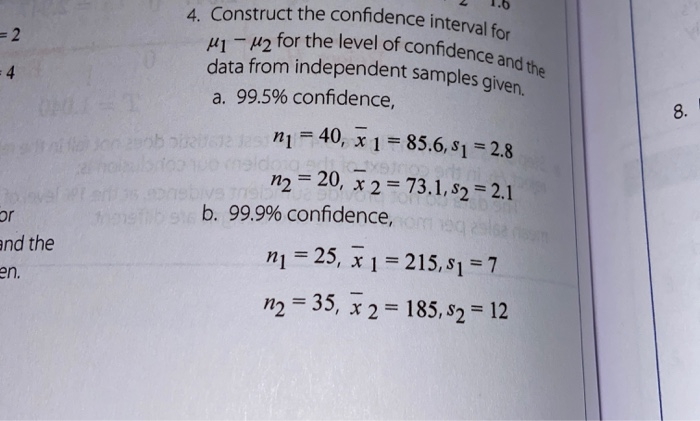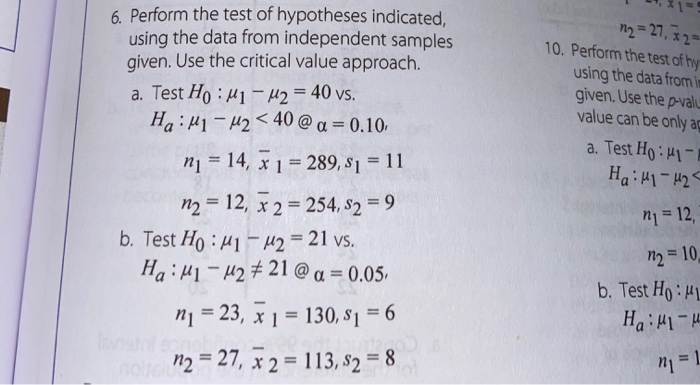= 2 2 1.6 4. Construct the confidence interval for u-u2 for the level of confiden data from independent samples give a. 99.5% confidence, of confidence and the - 4 bo n i= 40, x 1 = 85.6, 8, = 28 n2 = 20, x 2 = 73.1,82 = 2.1 bivs b. 99.9% confidence, ni = 25, x 1 = 215,81 = 7 n2 = 35, x 2 = 185,82 = 12 and the en.
n2 = 27, *2= 10. Perform the test of hy using the data from it given. Use the p-valu value can be only a a. Test Ho: 41- Haili-42- 6. Perform the test of hypotheses indicated, using the data from independent samples given. Use the critical value approach. a. Test Ho: M1 - U2 = 40 vs. He:M1 - 42<40 @ a = 0.10, ni = 14, x 1 = 289, S1 = 11 n2 = 12, x 2 = 254, 82 = 9 b. Test H:41 - uz = 21 vs. Ha: 41-42 # 21 @ a = 0.05, n1 = 23, x 1 = 130,81 = 6 o n2 = 27, x 2 = 113, 82 = 8 ni = 12, m2 = 10, b. Test Ho: M1 Ha:M1-4 n = 1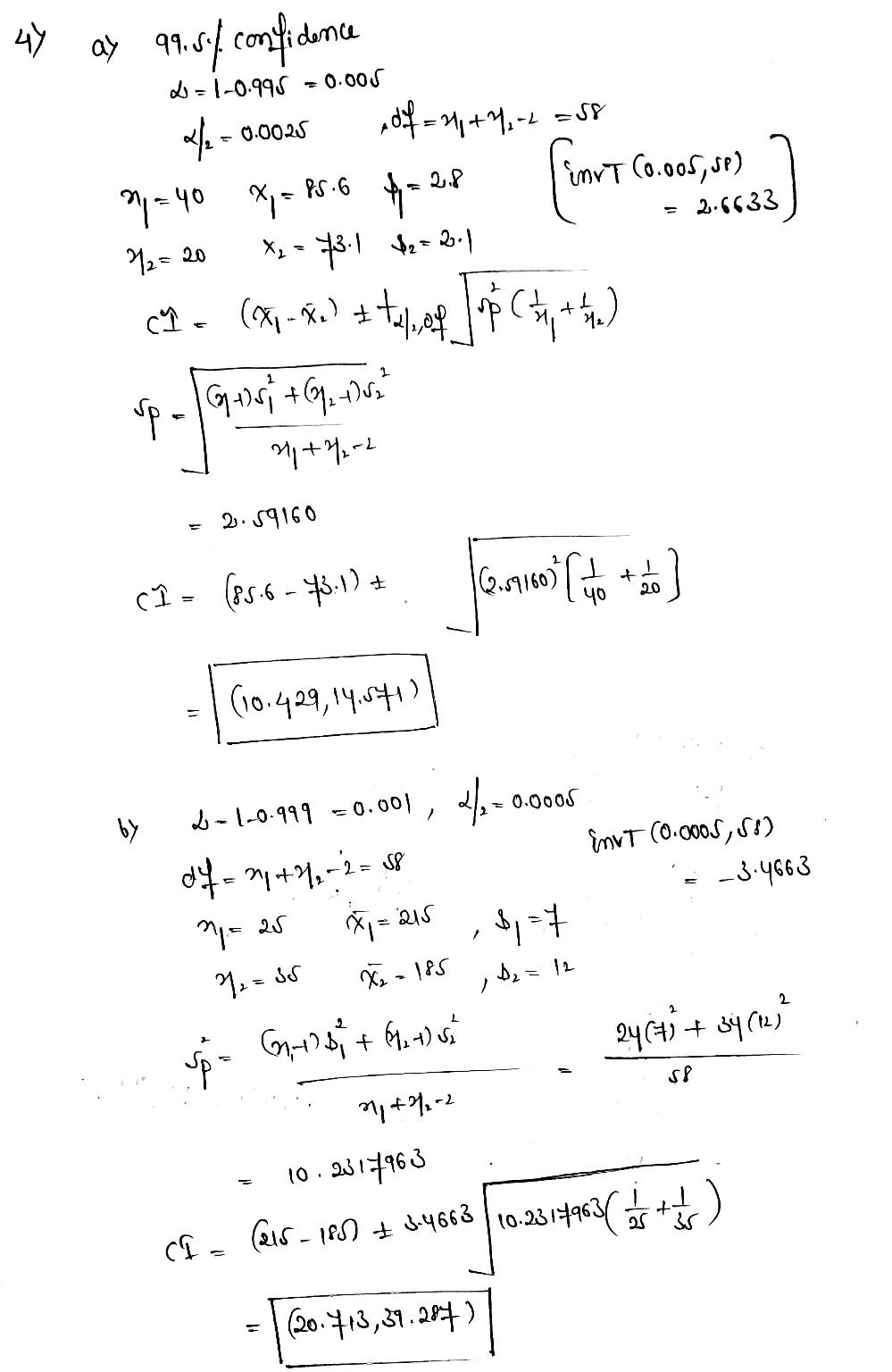##### Add Answer to: answer 4 and 6 = 2 2 1.6 4. Construct the confidence interval for u-u2 for...
Similar Homework Help Questions
• ### Perform the test of hypotheses indicated, using the data from independent samples given. Use the critical...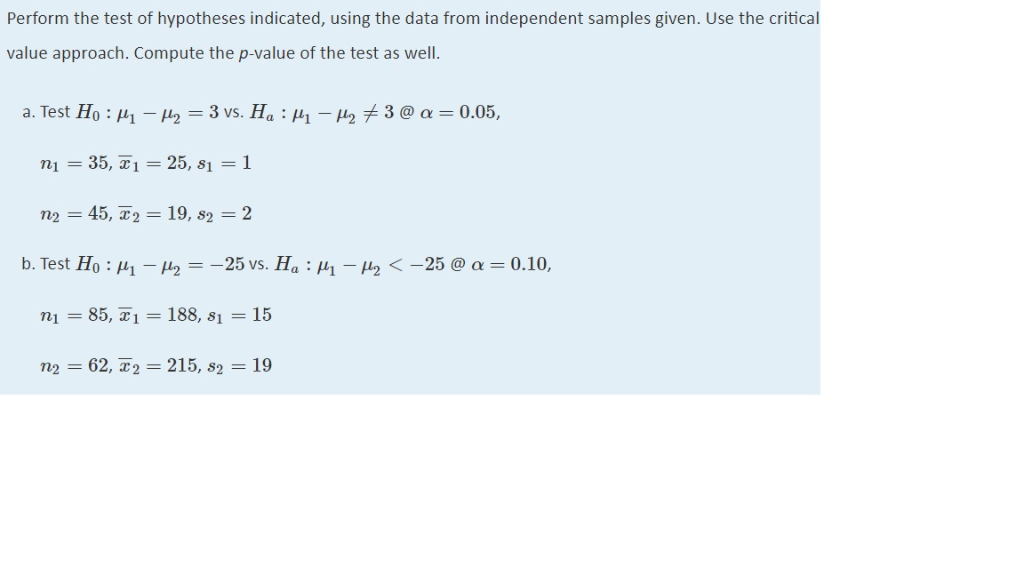Perform the test of hypotheses indicated, using the data from independent samples given. Use the critical value approach. Compute the p-value of the test as well α. Test Ho : μι-μ2 = 3 vs. Ha : μι-μ2メ 3 @ α = 0.05 , ni = 35, z i = 25, si = 1 ,S2 b.Test Ho : μι-ㄣ--25 vs. Ha : μι-μ2 <-25 @ α = 0.10. ni = 85, 2:1 = 188, 81 = 15 n2 = 62,-2-2 15,...

• ### 1. Consider the hypothesis test Ho: Mi=u2 against Hj: uit U2. Suppose that sample sizes are...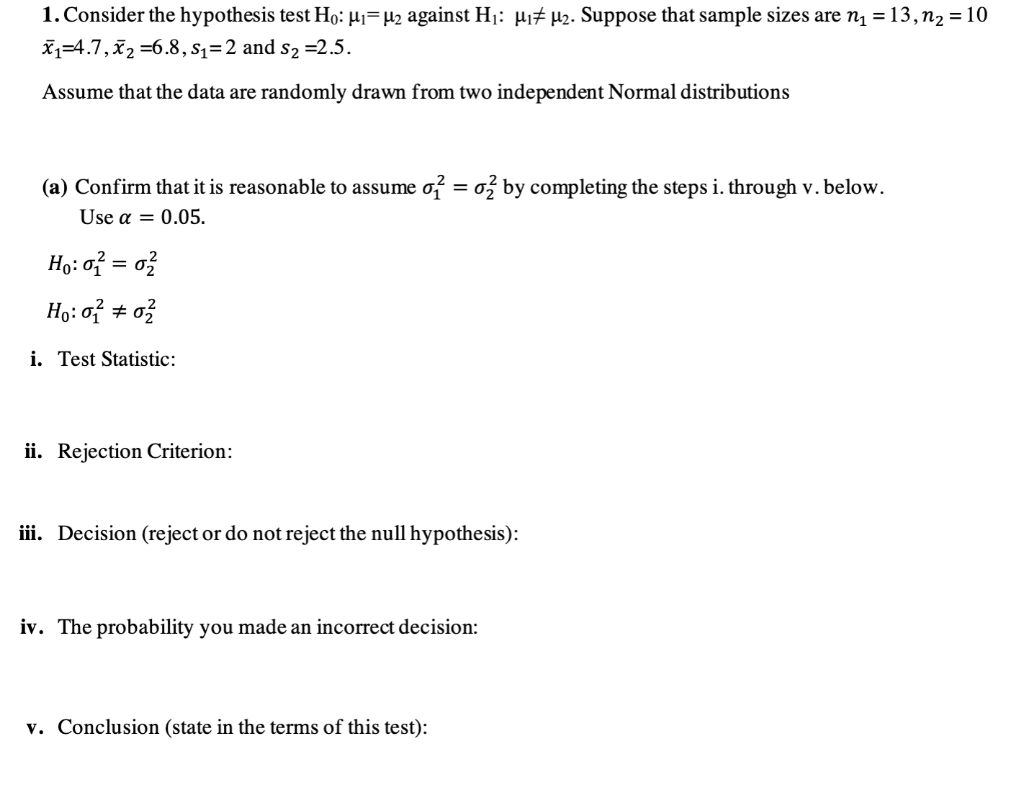1. Consider the hypothesis test Ho: Mi=u2 against Hj: uit U2. Suppose that sample sizes are ni = 13, n2 = 10 #1=4.7, X 2 =6.8, s1=2 and s2 =2.5. Assume that the data are randomly drawn from two independent Normal distributions (a) Confirm that it is reasonable to assume o? = ož by completing the steps i. through v. below. Use a = 0.05. Ho: 0 = ož Ho: 02 i. Test Statistic: ü. Rejection Criterion: iii. Decision (reject...

• ### Please help calculate the p value and confidence interval. Thanks! The confidence interval is: | Treatment...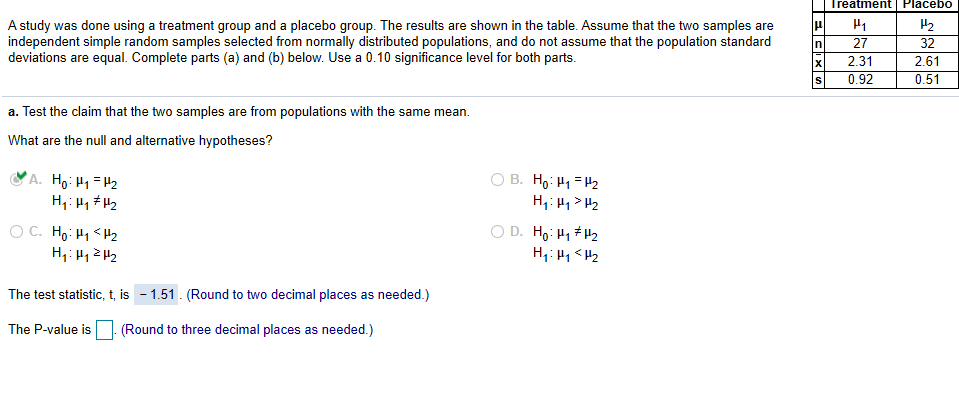Please help calculate the p value and confidence interval. Thanks! The confidence interval is: | Treatment Placebo A study was done using a treatment group and a placebo group. The results are shown in the table. Assume that the two samples are independent simple random samples selected from normally distributed populations, and do not assume that the population standard deviations are equal. Complete parts (a) and (b) below. Use a 0.10 significance level for both parts. XIF x s 27...

• ### Problem V. (9 points) Answer following questions. 1. (2 points) Suppose we construct a 90%-confidence interval...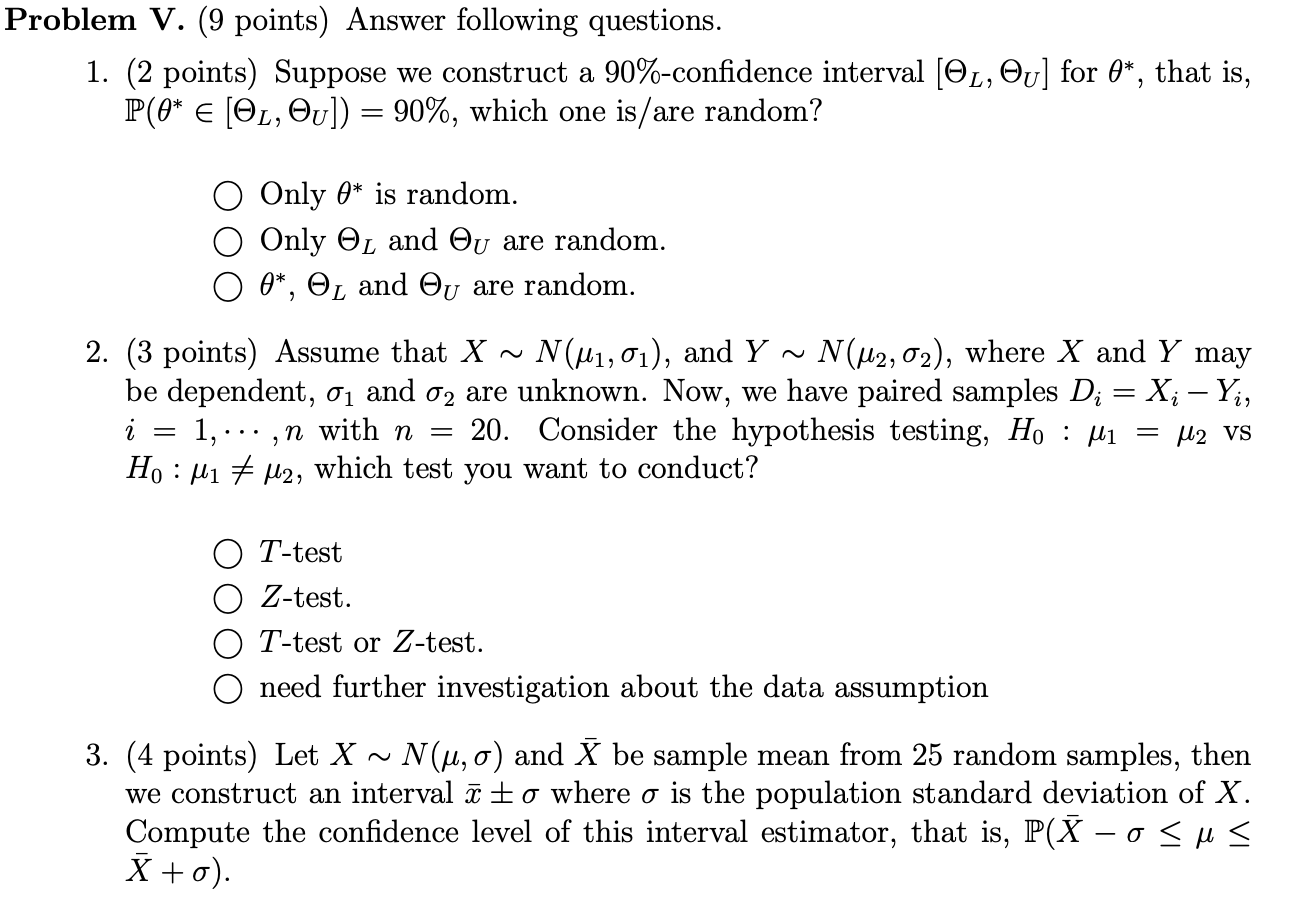Problem V. (9 points) Answer following questions. 1. (2 points) Suppose we construct a 90%-confidence interval [OL, Ou] for 0*, that is, P(0* E [OĽ, Ov]) = 90%, which one is/are random? O Only A* is random. Only and Ou are random. 0*, OL and Ou are random. 2. (3 points) Assume that X ~ N(M1,01), and Y ~ N(M2,02), where X and Y may be dependent, 01 and 02 are unknown. Now, we have paired samples Di = X;...

• ### Consider the hypothesis test Ho: Mi = U2 against HL : M1 <H2 with known variances...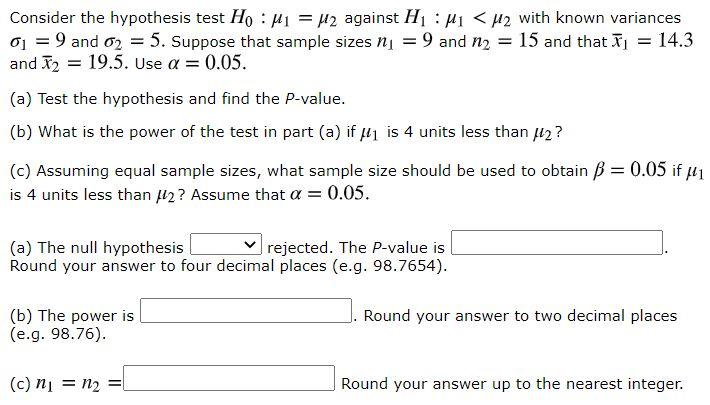Consider the hypothesis test Ho: Mi = U2 against HL : M1 <H2 with known variances a = 9 and 62 = 5. Suppose that sample sizes nj = 9 and n2 = 15 and that I = 14.3 and 12 = 19.5. Use a = 0.05. (a) Test the hypothesis and find the P-value. (b) What is the power of the test in part (a) if H1 is 4 units less than 2? (c) Assuming equal sample sizes, what...

• ### Problem V. (9 points) Answer following questions. 1. (2 points) Suppose we construct a 90%-confidence interval...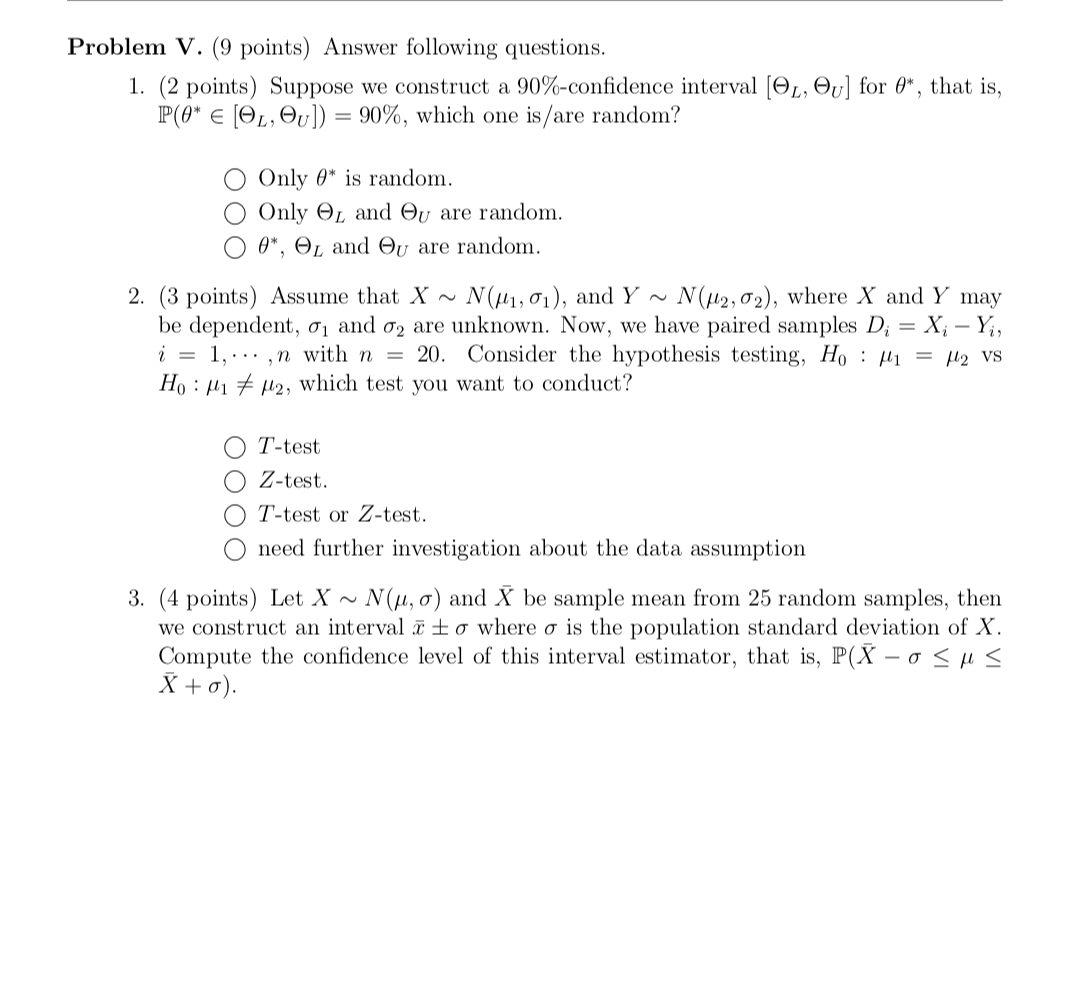Problem V. (9 points) Answer following questions. 1. (2 points) Suppose we construct a 90%-confidence interval (OL, Ov] for 4*, that is, P(@* E (OL, Ov]) = 90%, which one is/are random? Only 0* is random. Only OL and Ou are random. 0*, L and Ou are random. 2. (3 points) Assume that X ~ N(41,01), and Y ~ N(112,02), where X and Y may be dependent, 01 and 02 are unknown. Now, we have paired samples Di = Xi...

• ### 1. Consider the hypothesis test Ho: M1= H2 against Hj: Mit u2. Suppose that sample sizes...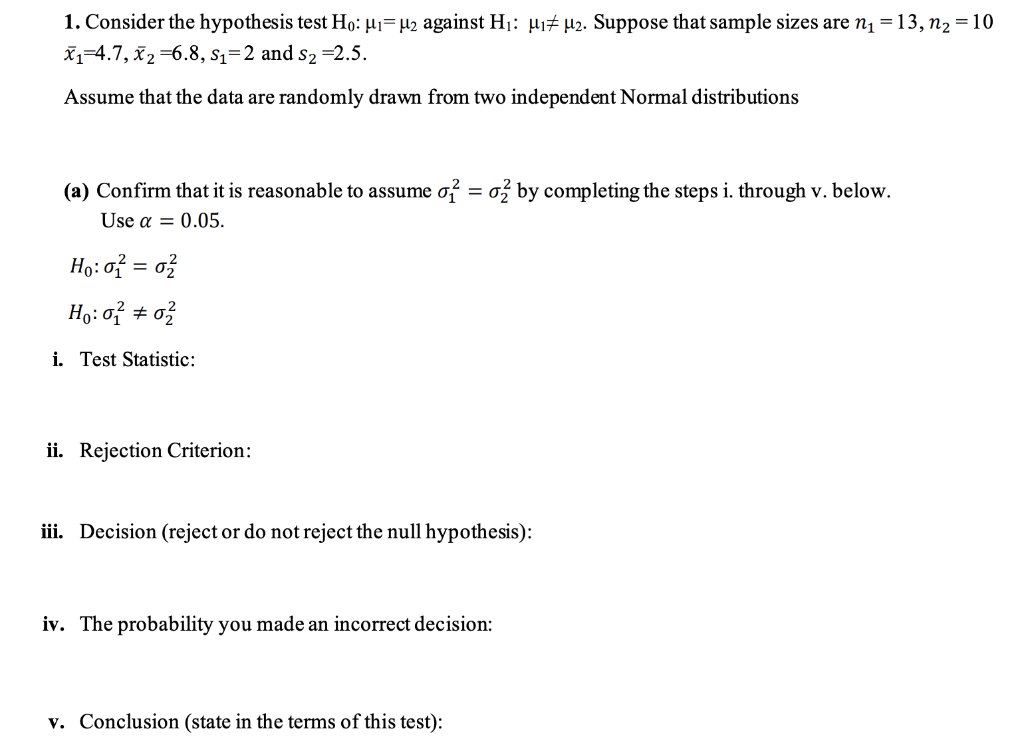1. Consider the hypothesis test Ho: M1= H2 against Hj: Mit u2. Suppose that sample sizes are ni = 13, N2 = 10 ži=4.7, X2 =6.8, S1=2 and s2 =2.5. Assume that the data are randomly drawn from two independent Normal distributions (a) Confirm that it is reasonable to assume o = ož by completing the steps i. through v. below. Use a = 0.05. Ho: 01 = ož Họ: 0 + 03 i. Test Statistic: ii. Rejection Criterion: iii....

• ### Problem V. (9 points) Answer following questions. 1. (2 points) Suppose we construct a 90%-confidence interval...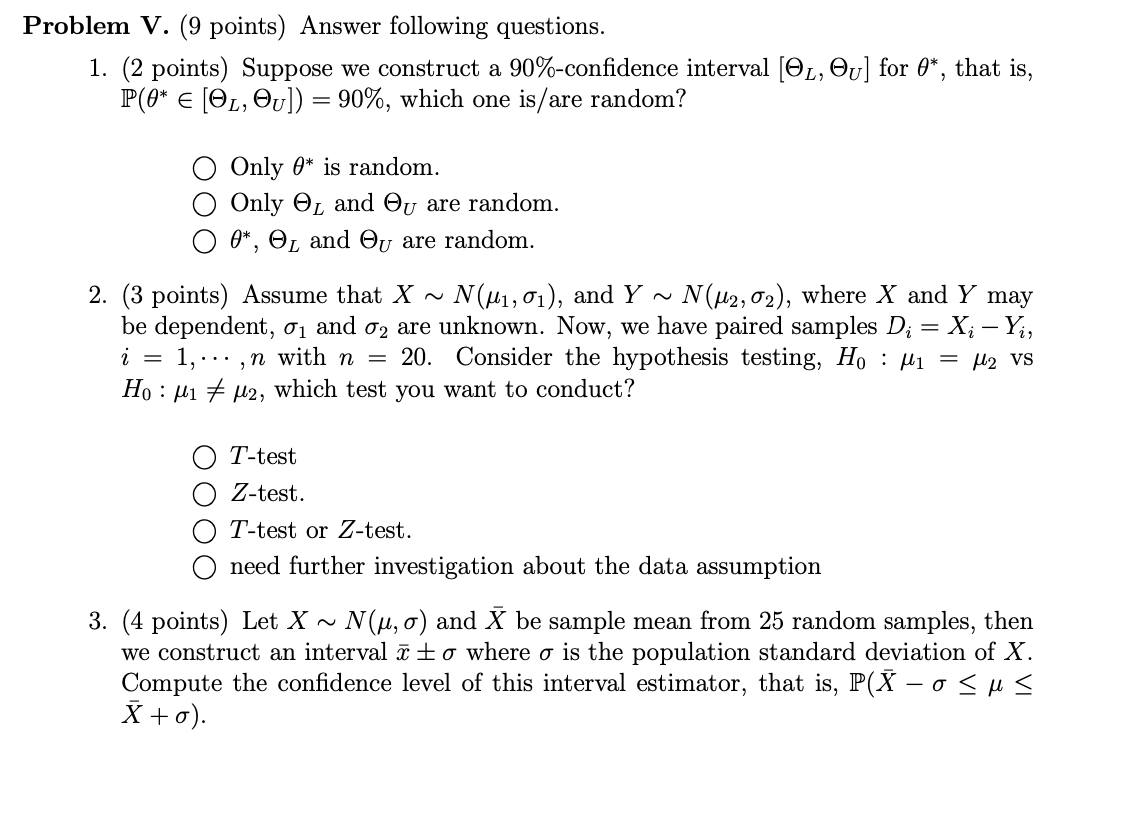Problem V. (9 points) Answer following questions. 1. (2 points) Suppose we construct a 90%-confidence interval [OL, Ou] for 4*, that is, P(0* E [OL, Ou]) = 90%, which one is/are random? Only O* is random. Only OL and Ou are random. 0*, OL and Ou are random. 2. (3 points) Assume that X ~ N(41,01), and Y ~ N(M2,02), where X and Y may be dependent, 01 and 02 are unknown. Now, we have paired samples Di = Xi...

• ### the screen below shows the 95% confidence interval for (u1,u2) what does the interval suggest about...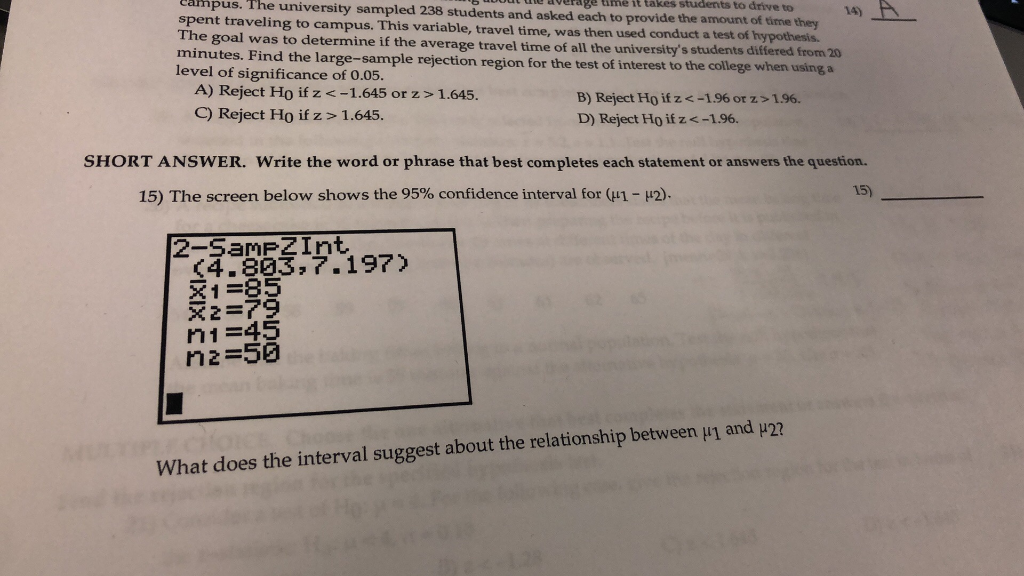the screen below shows the 95% confidence interval for (u1,u2) what does the interval suggest about the relationship between u1 and u2? Cafnpus. The university sampled 238 students and asked i takes students to drive to 14) A spent traveling to campus. This variable, travel time, was tto provide the amount of time they used conduct a test of hypothesis. The goal was to determine if the average travel time of all the tniversity's students differed from 20 minutes. Find...

• ### Chapter 10 Section 1 Additional Problem 1 Consider the hypothesis test Ho : M1 = up...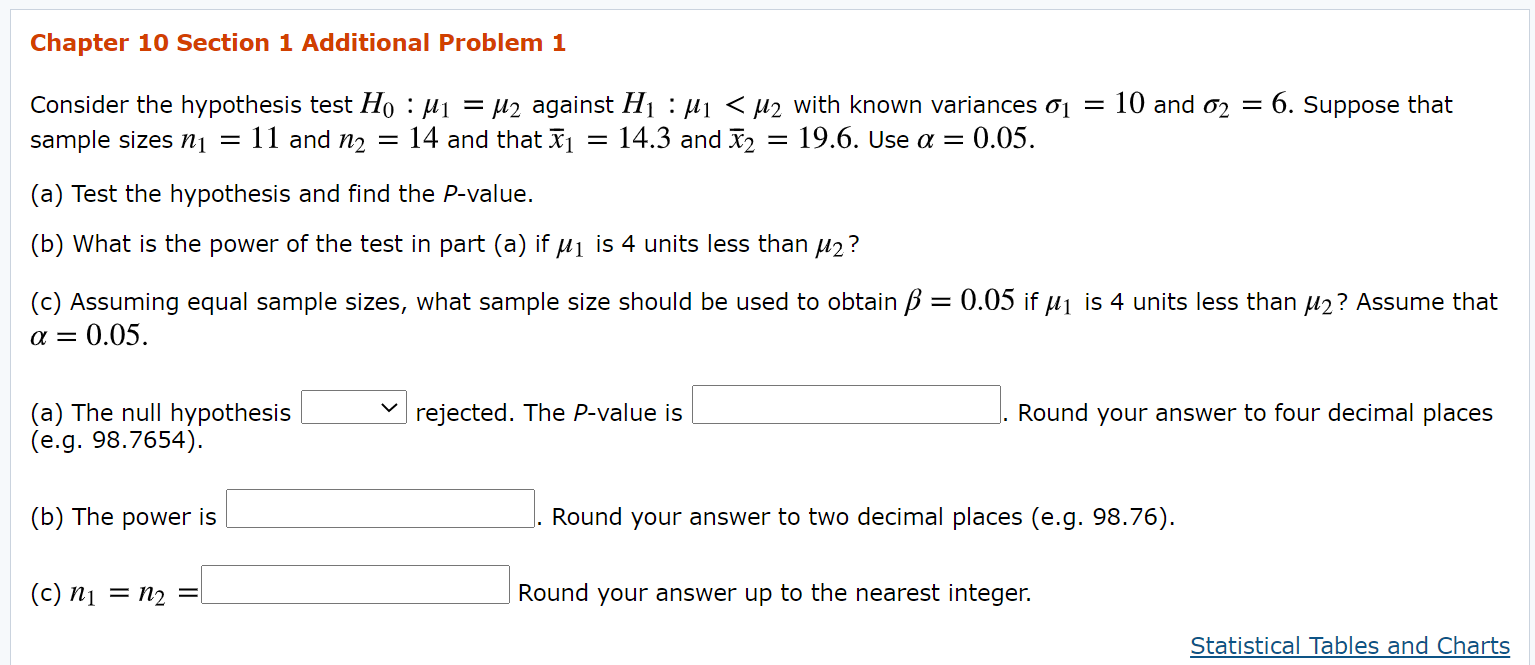Chapter 10 Section 1 Additional Problem 1 Consider the hypothesis test Ho : M1 = up against H1 : M1 < u2 with known variances 01 = 10 and 02 = - 6. Suppose that sample sizes ni = 11 and n2 = 14 and that ži = 14.3 and 12 = 19.6. Use a = 0.05. (a) Test the hypothesis and find the P-value. (b) What is the power of the test in part (a) if ui is 4...

Free Homework App